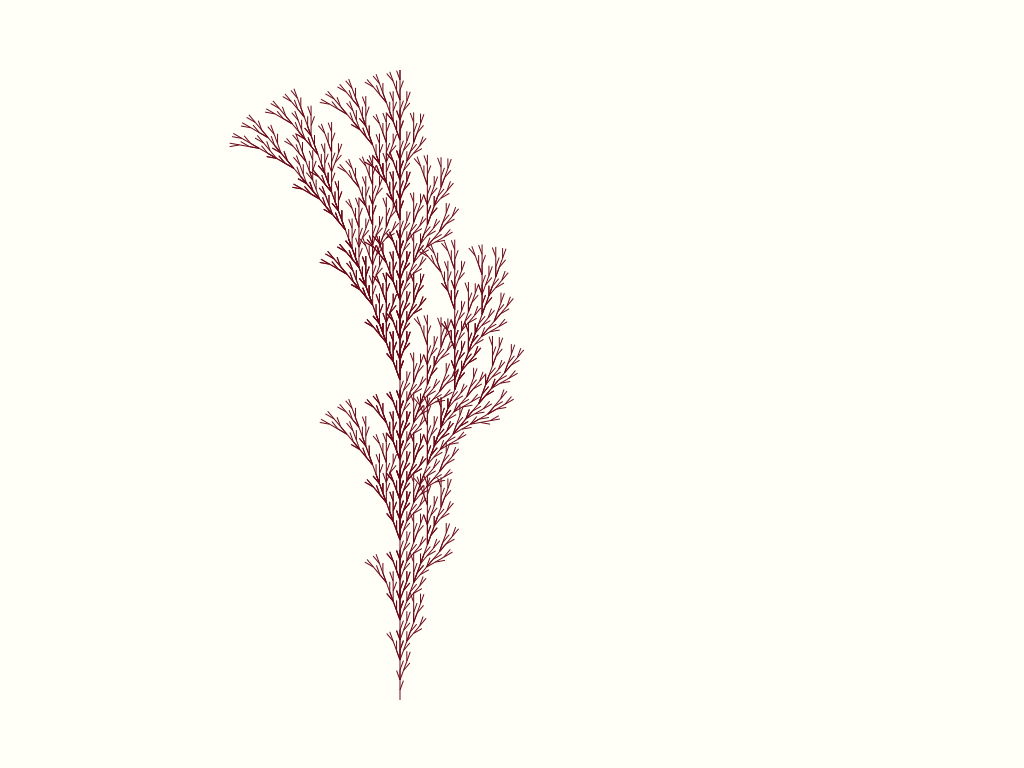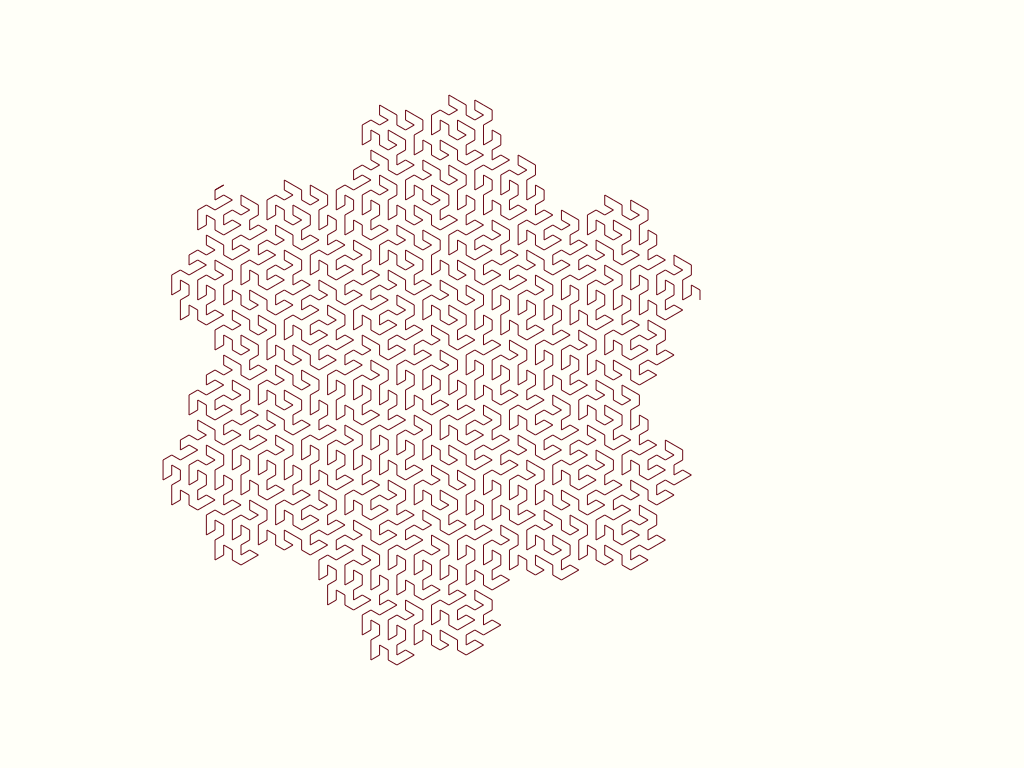# Out of intense complexities intense simplicities emerge…

### — A TypeScript implementation of L-systems

#### 2021-01-01

In the first chapter of The Algorithmic Beauty of Plants, the authors describe a set of related systems named L-systems after one of the authors, Lindenmayer. Using simple production rules, a L-system can produce advanced patterns, not so different from the visual patterns of plants. This is my implementation.A tree generated using a L-system. Starting with a `F`, and `F[+F]F[-F][F]` as substitution rule, recursively called 5 generations.

These are some signs that describe a potential condition.

``F-F-F-F``

When transiting from a state to another, then, according to a set of transformation rules, every F would be replaced with the following characters.

``FF-F--F-F``

All `-` characters are skipped.

So, this is the current condition,

``F-F-F-F``

The first visit to this initial axiom, would generate the first generation.

``FF-F--F-FFF-F--F-FFF-F--F-FFF-F--F-F``

A second would transform to:

``````FF-F--F-FFF-F--F-FFF-F--F-FFF-F--F-FFF-F--F-FFF-F--F-FFF-F--F-FFF-F--F-FFF-
F--F-FFF-F--F-FFF-F--F-FFF-F--F-FFF-F--F-FFF-F--F-FFF-F--F-FFF-F--F-FFF-F--F
-FFF-F--F-FFF-F--F-FFF-F--F-F``````

For each generation, the pattern would be more advanced.

There are also a set of rules for how to react to a condition, how each sign should be interpreted as a command and exectuted.

Assume a two-dimensional plane and a turtle walking about. In addition to a position, the turtle has a direction. Here is a set of rules that indicates how the turtle reacts to characters, a set of instructions for how the turtle navigates the plane. The turtle parses each character as an instruction:

``````F → forward and fill the position with a color
f → forward, but do not fill the position
+ → turn 90 degrees to the left
- → turn 90 degrees to the right``````

What happens when the turtle follows instructions `F-F-F-F`? If the turtle initially is directed to the north and walks forward it goes to the north, turns to the right, continues forward, heading east, turns, etc. until the turtle finally arrives at the same position from which it originated. The turtle’s “trace” forms a square.

The more times one state advances to another, the more advanced the pattern becomes. From the kind of flatness we experience when seeing simple geometric forms, the turtle moves — parsing and executing instructions — forming shapes like this Gosper Curve,Two sets of rules: One set of rules takes a number of characters, visits each character, and transforms it, given that the character is covered in the set of rules. A larger set of characters are returned from the transformation. The second set of rules is a list of instructions, rules for how to use the characters, how to execute them. From potentiality we achieve actuality and side-effects.

All patterns are constructed by repetition, and deepening. A larger pattern is similar to, and a repetition of, a smaller pattern. All such patterns are regular in an irregular way, dwelling in the borders between something finite and infinite.

My implementation uses TypeScript, and have utilized a functional style aiming for readability, not performance.

I made a function, `makeLSystem`, which takes a number of generations to be produced, an initial axiom, and a set of transformation rules.

If `makeLSystem` does not receive more than 0 generations, it returns the axiom that was taken. No validation of the characters’ authenticity is done because this function does not know what characters have meaning in the set of instructions.

Until the generation of transformations wanted has been completed, `makeLSystem` is called recursively, for each generation producing a new, transformed axiom.

``````type Axiom = string;

type LRules = Map<Axiom, Axiom>;

type Instruction = string;

const makeLSystem = (
generations = 0,
axiom:Axiom = '',
lrules:LRules
):Instruction => {
if(generations === 0) return axiom;
const mLS = (generation = 0, newAxiom:Axiom = ''):Axiom =>
generation === generations
? newAxiom
: generation === 0
? mLS(generation + 1, makeGeneration(axiom, lrules))
: mLS(generation + 1, makeGeneration(newAxiom, lrules));
return mLS();
};``````

`makeGeneration` takes a generations current axiom, the set of rules and return a new axiom after folding it using `nextGeneration`. If the character examined in `nextGeneration` is included in the set of rules, the character is replaced according to the taken rules, replacing the character with the character(s) associated with it. This continues until there are no characters left to be examined. A set of instructions are returned.

``````const nextGeneration = (rules:LRules) => (
acc:Axiom,
str:Axiom
) => acc.concat(rules.get(str) ?? str);

const makeGeneration = (prevGenerations:Axiom, rules:LRules) =>
prevGenerations
.split('')
.reduce(nextGeneration(rules), '');``````

How does this relate to the set of rules used for instructions? Let’s look at the rules of for the Pentadendrite. For each `F`, `F-F+F+FF-F-F+F`.

``````const pentadendrite = new Map()
.set('F', 'F-F-F++F+F-F');``````

The Pentadendrite starts with `F`, uses `F-F-F++F+F-F` as substitution rule, recursively called 4 generations.